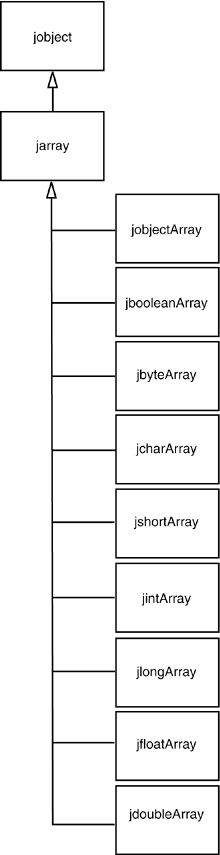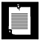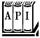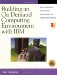# Accessing Array Elements

All array types of the Java programming language have corresponding C types, as shown in Table 11-2.

##### Table 11-2. Correspondence Between Java Array Types and C Types

Java Type

C Type

boolean[]

jbooleanArray

byte[]

jbyteArray

char[]

jcharArray

int[]

jintArray

short[]

jshortArray

long[]

jlongArray

float[]

jfloatArray

double[]

jdoubleArray

Object[]

jobjectArray

The type jarray denotes a generic array.

C++ NOTEIn C, all these array types are actually type synonyms of jobject. In C++, however, they are arranged in the inheritance hierarchy shown in Figure 11-2.

##### Figure 11-2. Inheritance hierarchy of array typesThe GetArrayLength function returns the length of an array.

` jarray array = . . .; jsize length = (*env)->GetArrayLength(env, array); `

How you access elements in the array depends on whether the array stores objects or a primitive type (bool, char, or a numeric type). You access elements in an object array with the GetObjectArrayElement and SetObjectArrayElement methods.

` jobjectArray array = . . .; int i, j; jobject x = (*env)->GetObjectArrayElement(env, array, i); (*env)->SetObjectArrayElement(env, array, j, x); `

While simple, this approach is also clearly inefficient; you want to be able to access array elements directly, especially when doing vector and matrix computations.

The GetXxxArrayElements function returns a C pointer to the starting element of the array. As with ordinary strings, you must remember to call the corresponding ReleaseXxxArrayElements function to tell the virtual machine when you no longer need that pointer. Here, the type Xxx must be a primitive type, that is, not Object. You can then read and write the array elements directly. However, since the pointer may point to a copy, any changes that you make are guaranteed to be reflected in the original array only when you call the corresponding ReleaseXxxArrayElements function!

NOTEYou can find out if an array is a copy by passing a pointer to a jboolean variable as the third parameter to a GetXxxArrayElements method. The variable is filled with JNI_TRUE if the array is a copy. If you aren't interested in that information, just pass a NULL pointer.

Here is a code sample that multiplies all elements in an array of double values by a constant. We obtain a C pointer a into the Java array and then access individual elements as a[i].

` jdoubleArray array_a = . . .; double scaleFactor = . . .; double* a = (*env)->GetDoubleArrayElements(env, array_a, NULL); for (i = 0; i < (*env)->GetArrayLength(env, array_a); i++)    a[i] = a[i] * scaleFactor; (*env)->ReleaseDoubleArrayElements(env, array_a, a, 0); `

Whether the virtual machine actually copies the array depends on how it allocates arrays and does its garbage collection. Some "copying" garbage collectors routinely move objects around and update object references. That strategy is not compatible with "pinning" an array to a particular location, because the collector cannot update the pointer values in native code.

NOTEIn the Sun JVM implementation, boolean arrays are represented as packed arrays of 32-bit words. The GetBooleanArrayElements method copies them into unpacked arrays of jboolean values.

To access just a few elements of a large array, use the GetXxxArrayRegion and SetXxxArrayRegion methods that copy a range of elements from the Java array into a C array and back.

You can create new Java arrays in native methods with the NewXxxArray function. To create a new array of objects, you specify the length, the type of the array elements, and an initial element for all entries (typically, NULL). Here is an example.

` jclass class_Employee = (*env)->FindClass(env, "Employee"); jobjectArray array_e = (*env)->NewObjectArray(env, 100, class_Employee, NULL); `

Arrays of primitive types are simpler. You just supply the length of the array.

` jdoubleArray array_d = (*env)->NewDoubleArray(env, 100); `

The array is then filled with zeroes.

NOTEJDK 1.4 adds three methods to the JNI API:` jobject NewDirectByteBuffer(JNIEnv* env, void* address, jlong capacity) void* GetDirectBufferAddress(JNIEnv* env, jobject buf) jlong GetDirectBufferCapacity(JNIEnv* env, jobject buf) `Direct buffers are used in the java.nio package to support more efficient input/output operations and to minimize the copying of data between native and Java arrays.

``

` Manipulating Java Arrays in C Code `

• jsize GetArrayLength(JNIEnv *env, jarray array)

returns the number of elements in the array.

 Parameters: env The JNI interface pointer array The array object

• jobject GetObjectArrayElement(JNIEnv *env, jobjectArray array, jsize index)

returns the value of an array element.

 Parameters: env The JNI interface pointer array The array object index The array offset

• void SetObjectArrayElement(JNIEnv *env, jobjectArray array, jsize index, jobject value)

sets an array element to a new value.

 Parameters: env The JNI interface pointer array The array object index The array offset value The new value

• Xxx* GetXxxArrayElements(JNIEnv *env, jarray array, jboolean* isCopy)

yields a C pointer to the elements of a Java array. The field type Xxx is one of Boolean, Byte, Char, Short, Int, Long, Float, or Double. The pointer must be passed to ReleaseXxxArrayElements when it is no longer needed.

 Parameters: env The JNI interface pointer array The array object isCopy Is either NULL or points to a jboolean that is filled with JNI_TRUE if a copy is made; with JNI_FALSE otherwise

• void ReleaseXxxArrayElements(JNIEnv *env, jarray array, Xxx elems[], jint mode)

notifies the virtual machine that a pointer obtained by GetXxxArrayElements is no longer needed.

 Parameters: env The JNI interface pointer array The array object elems The pointer to the array elements that is no longer needed mode 0 = free the elems buffer after updating the array elements JNI_COMMIT = do not free the elems buffer after updating the array elements JNI_ABORT = free the elems buffer without updating the array elements

• void GetXxxArrayRegion(JNIEnv *env, jarray array, jint start, jint length, Xxx elems[])

copies elements from a Java array to a C array. The field type Xxx is one of Boolean, Byte, Char, Short, Int, Long, Float, or Double.

 Parameters: env The JNI interface pointer array The array object start The starting index length The number of elements to copy elems The C array that holds the elements

• void SetXxxArrayRegion(JNIEnv *env, jarray array, jint start, jint length, Xxx elems[])

copies elements from a C array to a Java array. The field type Xxx is one of Boolean, Byte, Char, Short, Int, Long, Float, or Double.

 Parameters: env The JNI interface pointer array The array object start The starting index length The number of elements to copy elems The C array that holds the elementsBuilding an On Demand Computing Environment with IBM: How to Optimize Your Current Infrastructure for Today and Tomorrow (MaxFacts Guidebook series)
ISBN: 193164411X
EAN: 2147483647
Year: 2003
Pages: 156
Authors: Jim Hoskins